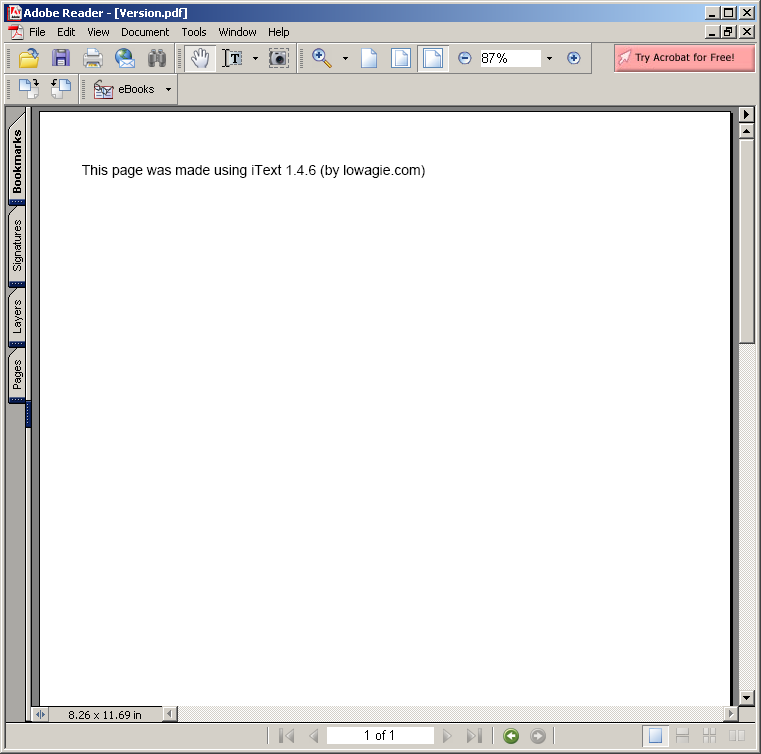# implements the LZF lossless data compression algorithm : Algorithms « Collections Data Structure « Java

implements the LZF lossless data compression algorithm

```
/*
* Copyright 2004-2010 H2 Group. Multiple-Licensed under the H2 License,
* Version 1.0, and under the Eclipse Public License, Version 1.0
* Copyright (c) 2000-2005 Marc Alexander Lehmann <schmorp@schmorp.de>
* Copyright (c) 2005 Oren J. Maurice <oymaurice@hazorea.org.il>
*
* Redistribution and use in source and binary forms, with or without
* modification, are permitted provided that the following conditions are met:
*
*   1.  Redistributions of source code must retain the above copyright notice,
*       this list of conditions and the following disclaimer.
*
*   2.  Redistributions in binary form must reproduce the above copyright
*       notice, this list of conditions and the following disclaimer in the
*       documentation and/or other materials provided with the distribution.
*
*   3.  The name of the author may not be used to endorse or promote products
*       derived from this software without specific prior written permission.
*
* THIS SOFTWARE IS PROVIDED BY THE AUTHOR ''AS IS'' AND ANY EXPRESS OR IMPLIED
* WARRANTIES, INCLUDING, BUT NOT LIMITED TO, THE IMPLIED WARRANTIES OF
* MERCHANTABILITY AND FITNESS FOR A PARTICULAR PURPOSE ARE DISCLAIMED.  IN NO
* EVENT SHALL THE AUTHOR BE LIABLE FOR ANY DIRECT, INDIRECT, INCIDENTAL,
* SPECIAL, EXEMPLARY, OR CONSEQUENTIAL DAMAGES (INCLUDING, BUT NOT LIMITED TO,
* PROCUREMENT OF SUBSTITUTE GOODS OR SERVICES; LOSS OF USE, DATA, OR PROFITS;
* OR BUSINESS INTERRUPTION) HOWEVER CAUSED AND ON ANY THEORY OF LIABILITY,
* WHETHER IN CONTRACT, STRICT LIABILITY, OR TORT (INCLUDING NEGLIGENCE OR
* OTHERWISE) ARISING IN ANY WAY OUT OF THE USE OF THIS SOFTWARE, EVEN IF ADVISED
* OF THE POSSIBILITY OF SUCH DAMAGE.
*/

//package com.hyk.proxy.common.rpc.extension.compress.lzf;

/**
* <p>
* This class implements the LZF lossless data compression algorithm. LZF is a
* Lempel-Ziv variant with byte-aligned output, and optimized for speed.
* </p>
* <p>
* Safety/Use Notes:
* </p>
* <ul>
* <li>Each instance should be used by a single thread only.</li>
* <li>The data buffers should be smaller than 1 GB.</li>
* <li>For performance reasons, safety checks on expansion are omitted.</li>
* <li>Invalid compressed data can cause an ArrayIndexOutOfBoundsException.</li>
* </ul>
* <p>
* The LZF compressed format knows literal runs and back-references:
* </p>
* <ul>
* <li>Literal run: directly copy bytes from input to output.</li>
* <li>Back-reference: copy previous data to output stream, with specified
* offset from location and length. The length is at least 3 bytes.</li>
* </ul>
*<p>
* The first byte of the compressed stream is the control byte. For literal
* runs, the highest three bits of the control byte are not set, the the lower
* bits are the literal run length, and the next bytes are data to copy directly
* into the output. For back-references, the highest three bits of the control
* byte are the back-reference length. If all three bits are set, then the
* back-reference length is stored in the next byte. The lower bits of the
* control byte combined with the next byte form the offset for the
* back-reference.
* </p>
*/
public final class CompressLZF implements Compressor {

/**
* The number of entries in the hash table. The size is a trade-off between
* hash collisions (reduced compression) and speed (amount that fits in CPU
* cache).
*/
private static final int HASH_SIZE = 1 << 14;

/**
* The maximum number of literals in a chunk (32).
*/
private static final int MAX_LITERAL = 1 << 5;

/**
* The maximum offset allowed for a back-reference (8192).
*/
private static final int MAX_OFF = 1 << 13;

/**
* The maximum back-reference length (264).
*/
private static final int MAX_REF = (1 << 8) + (1 << 3);

/**
* Hash table for matching byte sequences (reused for performance).
*/
private int[] cachedHashTable;

/**
* Return byte with lower 2 bytes being byte at index, then index+1.
*/
private static int first(byte[] in, int inPos) {
return (in[inPos] << 8) | (in[inPos + 1] & 255);
}

/**
* Shift v 1 byte left, add value at index inPos+2.
*/
private static int next(int v, byte[] in, int inPos) {
return (v << 8) | (in[inPos + 2] & 255);
}

/**
* Compute the address in the hash table.
*/
private static int hash(int h) {
return ((h * 2777) >> 9) & (HASH_SIZE - 1);
}

public int compress(byte[] in, int inLen, byte[] out, int outPos) {
int inPos = 0;
if (cachedHashTable == null) {
cachedHashTable = new int[HASH_SIZE];
}
int[] hashTab = cachedHashTable;
int literals = 0;
outPos++;
int future = first(in, 0);
while (inPos < inLen - 4) {
byte p2 = in[inPos + 2];
// next
future = (future << 8) + (p2 & 255);
int off = hash(future);
int ref = hashTab[off];
hashTab[off] = inPos;
if (ref < inPos
&& ref > 0
&& (off = inPos - ref - 1) < MAX_OFF
&& in[ref + 2] == p2
&& in[ref + 1] == (byte) (future >> 8)
&& in[ref] == (byte) (future >> 16)) {
// match
int maxLen = inLen - inPos - 2;
if (maxLen > MAX_REF) {
maxLen = MAX_REF;
}
if (literals == 0) {
// multiple back-references,
// so there is no literal run control byte
outPos--;
} else {
// set the control byte at the start of the literal run
// to store the number of literals
out[outPos - literals - 1] = (byte) (literals - 1);
literals = 0;
}
int len = 3;
while (len < maxLen && in[ref + len] == in[inPos + len]) {
len++;
}
len -= 2;
if (len < 7) {
out[outPos++] = (byte) ((off >> 8) + (len << 5));
} else {
out[outPos++] = (byte) ((off >> 8) + (7 << 5));
out[outPos++] = (byte) (len - 7);
}
out[outPos++] = (byte) off;
// move one byte forward to allow for a literal run control byte
outPos++;
inPos += len;
// Rebuild the future, and store the last bytes to the hashtable.
// Storing hashes of the last bytes in back-reference improves
// the compression ratio and only reduces speed slightly.
future = first(in, inPos);
future = next(future, in, inPos);
hashTab[hash(future)] = inPos++;
future = next(future, in, inPos);
hashTab[hash(future)] = inPos++;
} else {
// copy one byte from input to output as part of literal
out[outPos++] = in[inPos++];
literals++;
// at the end of this literal chunk, write the length
// to the control byte and start a new chunk
if (literals == MAX_LITERAL) {
out[outPos - literals - 1] = (byte) (literals - 1);
literals = 0;
// move ahead one byte to allow for the
// literal run control byte
outPos++;
}
}
}
// write the remaining few bytes as literals
while (inPos < inLen) {
out[outPos++] = in[inPos++];
literals++;
if (literals == MAX_LITERAL) {
out[outPos - literals - 1] = (byte) (literals - 1);
literals = 0;
outPos++;
}
}
// writes the final literal run length to the control byte
out[outPos - literals - 1] = (byte) (literals - 1);
if (literals == 0) {
outPos--;
}
return outPos;
}

public void expand(byte[] in, int inPos, int inLen, byte[] out, int outPos, int outLen) {
if (inPos < 0 || outPos < 0 || outLen < 0) {
throw new IllegalArgumentException();
}
do {
int ctrl = in[inPos++] & 255;
if (ctrl < MAX_LITERAL) {
// literal run of length = ctrl + 1,
ctrl++;
// copy to output and move forward this many bytes
System.arraycopy(in, inPos, out, outPos, ctrl);
outPos += ctrl;
inPos += ctrl;
} else {
// back reference
// the highest 3 bits are the match length
int len = ctrl >> 5;
// if the length is maxed, add the next byte to the length
if (len == 7) {
len += in[inPos++] & 255;
}
// minimum back-reference is 3 bytes,
// so 2 was subtracted before storing size
len += 2;

// ctrl is now the offset for a back-reference...
// the logical AND operation removes the length bits
ctrl = -((ctrl & 0x1f) << 8) - 1;

// the next byte augments/increases the offset
ctrl -= in[inPos++] & 255;

// copy the back-reference bytes from the given
// location in output to current position
ctrl += outPos;
if (outPos + len >= out.length) {
// reduce array bounds checking
throw new ArrayIndexOutOfBoundsException();
}
for (int i = 0; i < len; i++) {
out[outPos++] = out[ctrl++];
}
}
} while (outPos < outLen);
}

}

/**
* Each data compression algorithm must implement this interface.
*/
interface Compressor {

/**
* Compress a number of bytes.
*
* @param in the input data
* @param inLen the number of bytes to compress
* @param out the output area
* @param outPos the offset at the output array
* @return the end position
*/
int compress(byte[] in, int inLen, byte[] out, int outPos);

/**
* Expand a number of compressed bytes.
*
* @param in the compressed data
* @param inPos the offset at the input array
* @param inLen the number of bytes to read
* @param out the output area
* @param outPos the offset at the output array
* @param outLen the size of the uncompressed data
*/
void expand(byte[] in, int inPos, int inLen, byte[] out, int outPos, int outLen);

}

```

### Related examples in the same category

 1 Anagrams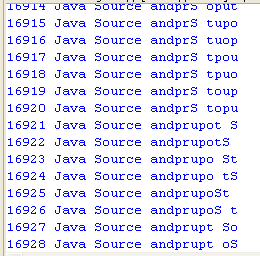2 Hanoi puzzle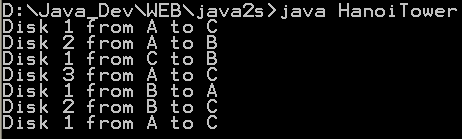3 Fibonacci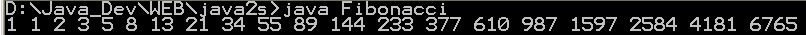4 Sieve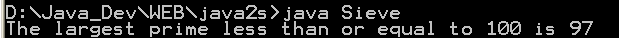5 Find connections using a depth-first search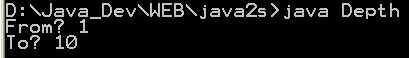6 Find connections using hill climbing. 7 Find optimal solution using least-cost 8 Find the lost keys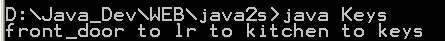9 Compute the area of a triangle using Heron's Formula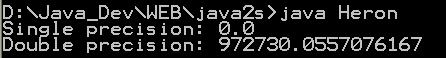10 Compute prime numbers 11 Print a table of fahrenheit and celsius temperatures 1 12 Print a table of fahrenheit and celsius temperatures 2 13 Print a table of Fahrenheit and Celsius temperatures 3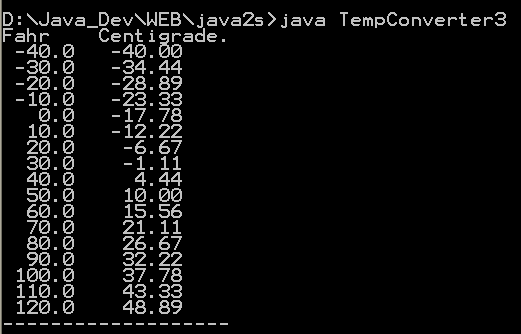14 Soundex - the Soundex Algorithm, as described by Knuth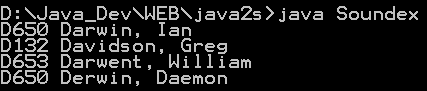15 A programmable Finite State Machine implementation. 16 An extendable Graph datastructure. 17 Utilities for flop (floating-point operation) counting. 18 LU Decomposition 19 Reverse Polish Notation 20 Permutator test 21 Linear Interpolation 22 Utility class for generating the k-subsets of the numbers 0 to n 23 Version